Students can download Maths Chapter 3 Algebra Ex 3.10 Questions and Answers, Notes, Samacheer Kalvi 9th Maths Guide Pdf helps you to revise the complete Tamilnadu State Board New Syllabus, helps students complete homework assignments and to score high marks in board exams.

## Tamilnadu Samacheer Kalvi 9th Maths Solutions Chapter 3 Algebra Ex 3.10

Question 1.
Draw the graph for the following:
(i) y = 2x
Solution:
When x = -2, y = 2 (-2) = -4
When x = 0, y = 2 (0) = 0
When x = 2, y = 2 (2) = 4
When x = 3, y = 2 (3) = 6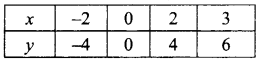Plot the points (-2, -4) (0, 0) (2, 4) and (3, 6) in the graph sheet we get a straight line.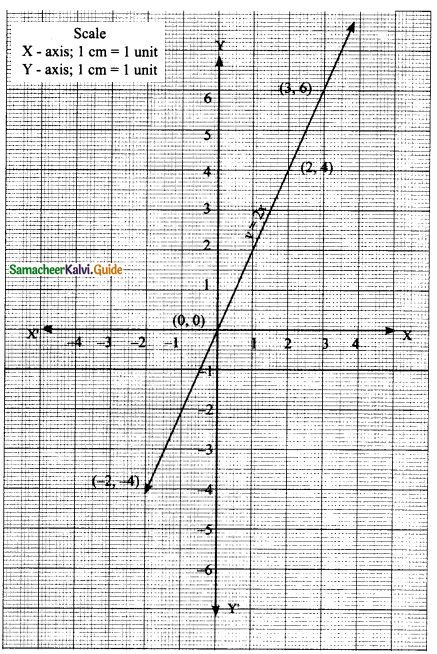(ii) y = 4x – 1
Solution:
When x = – 1; y = 4 (-1) -1 ⇒ y = -5
When x = 0; y = 4 (0) – 1 = 0 – 1 ⇒ y = -1
When x = 2; y = 4 (2) -1 = 8 – 1 ⇒ y = l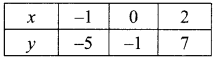Plot the points (-1, -5) (0, -1) and (2, 7) in the graph sheet we get a straight line. At the time of printing change the direction.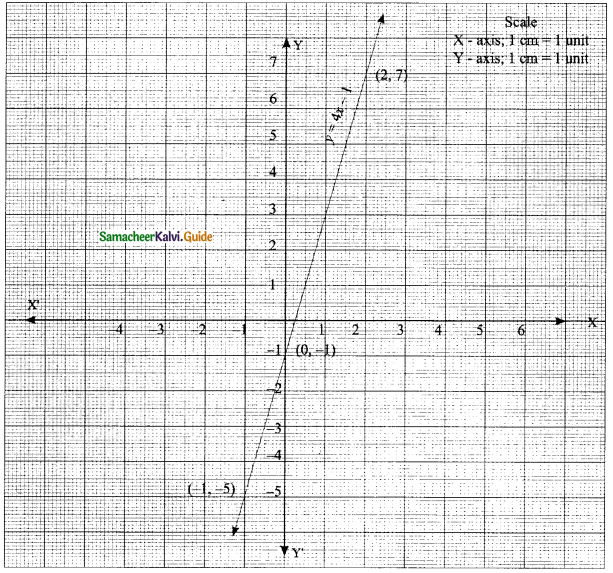(iii) y = ($$\frac{3}{2}$$)x + 3
Solution:
When x = -2;
y = $$\frac{3}{2}$$(-2) + 3
y = -3 + 3 = 0
when x = 0;
y = $$\frac{3}{2}$$(0) + 3
y = 3
when x = 2;
y = $$\frac{3}{2}$$(2) + 3
y = 3 + 3
= 6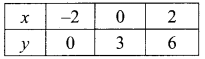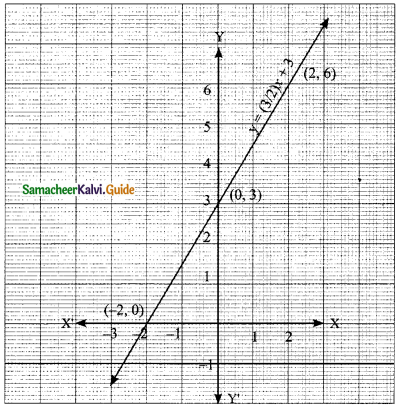Plot the points (-2, 0) (0, 3) and (2, 6) in the graph sheet we get a straight line.(iv) 3x + 2y = 14
Solution:
y = $$\frac{-3x+14}{2}$$
y = – $$\frac{3}{2}$$x + 7
when x = -2
y = –$$\frac{3}{2}$$(-2) + 7 = 10
when x = 0
y = –$$\frac{3}{2}$$(0) + 7 = 7
when x = 2
y = –$$\frac{3}{2}$$(2) + 7 = 4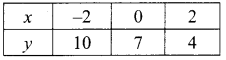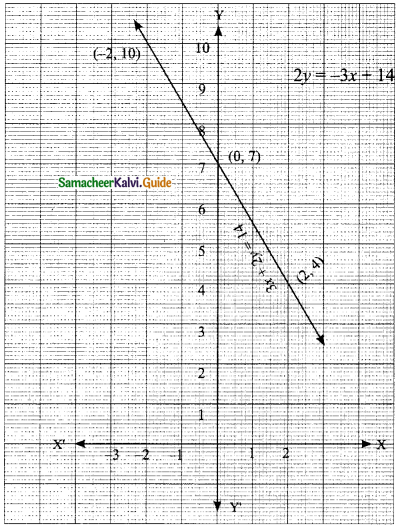Plot the points (-2, 10) (0, 7) and (2, 4) in the graph sheet we get a straight line.Question 2.
Solve graphically (i) x + y = 7, x – y = 3
Solution:
x + y = 7
y = 7 – x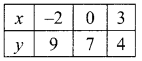Plot the points (-2, 9), (0, 7) and (3, 4) in the graph sheet
x – y = 3
-y = -x + 3
y = x – 3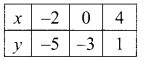Plot the points (-2, -5), (0, -3) and (4, 1) in the same graph sheet.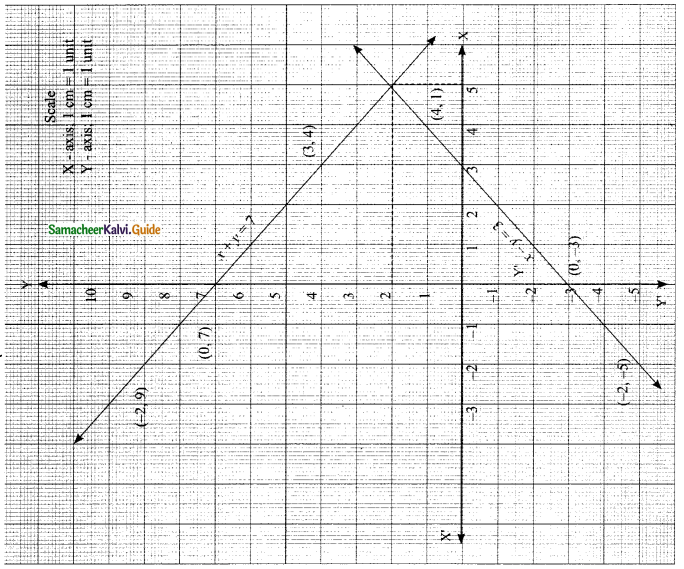The point of intersection is (5, 2) of lines (1) and (2).
The solution set is (5,2).(ii) 3x + 2y = 4; 9x + 6y – 12 = 0
Solution:
2y = -3x + 4
y = $$\frac{-3x+4}{2}$$
= $$\frac{-3}{2}$$x + 2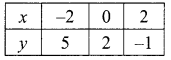Plot the points (-2, 5), (0, 2) and (2, -1) in the graph sheet
9x + 6y= 12 (÷3)
3x + 2y = 4
2y = $$\frac{-3x+4}{2}$$
= $$\frac{-3}{2}$$x + 2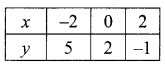Plot the points (-2, 5), (0, 2) and (2, -1) the same graph sheet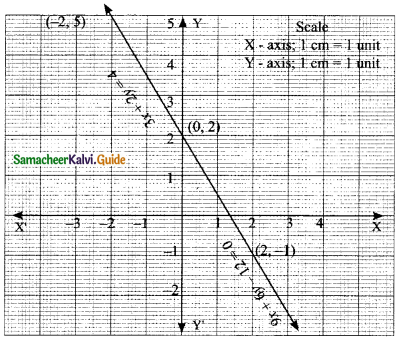Here both the equations are identical, but in different form.
Their solution is same.
This equations have an infinite number of solution.(iii) $$\frac{x}{2}$$ + $$\frac{y}{4}$$ = 1: $$\frac{x}{2}$$ + $$\frac{y}{4}$$ = 2
Solution:
$$\frac{x}{2}$$ + $$\frac{y}{4}$$ = 1
multiply by 4
2x + y = 4
y = -2x + 4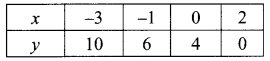Plot the points (-3, 10), (-1, 6), (0, 4) and (2, 0) in the graph sheet
$$\frac{x}{2}$$ + $$\frac{y}{4}$$ = 2
multiply by 4
2x + y = 8
y = -2x + 8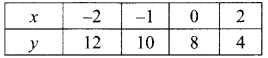Plot the points (-2, 12), (-1, 10), (0, 8) and (2, 4) in the same graph sheet.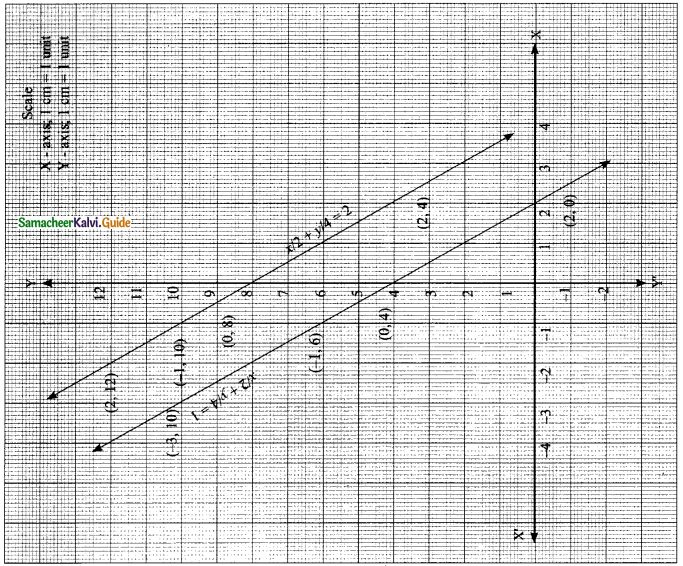The given two lines are parallel.
∴ They do not intersect a point.
∴ There is no solution.(iv) x – y = 0; y + 3 = 0
Solution:
x – y = 0
-y = -x
y = x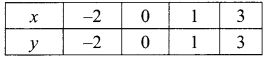Plot the points (-2, -2), (0, 0), (1, 1) and (3, 3) in the same graph sheet.
y + 3 = 0
y = -3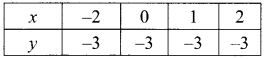Plot the points (-2, -3), (0, -3), (1, -3) and (2, -3) in the same graph sheet.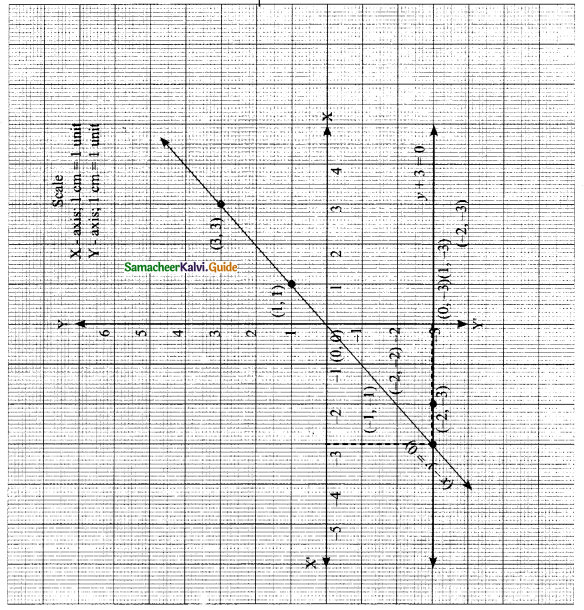The two lines l1 and l2 intersect at (-3, -3). The solution set is (-3, -3).(v) y = 2x + 1; 3x – 6 = 0
Solution:
y = 2x + 1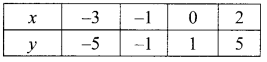Plot the points (-3, -5), (-1, -1), (0, 1) and (2, 5) in the graph sheet
3x – 6 = 0
y = -3x + 6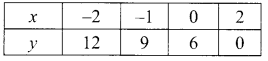Plot the points (-2, 12), (-1, 9), (0, 6) and (2, 0) in the same graph sheet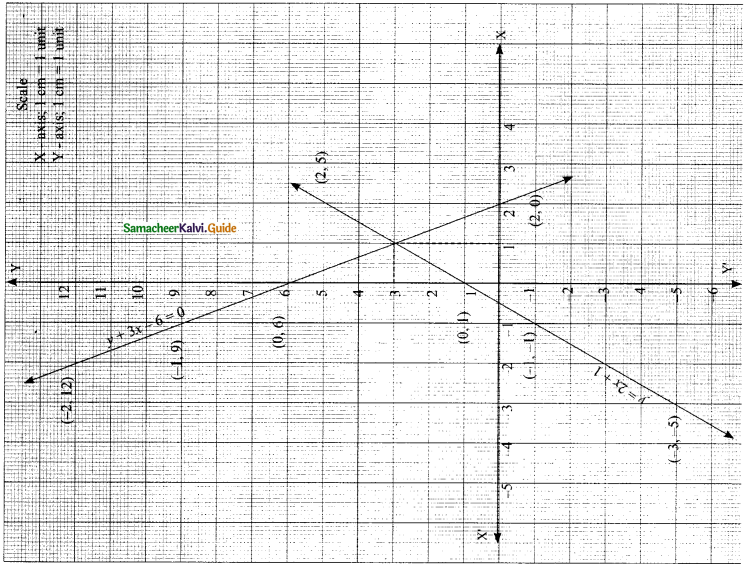The two lines l1 and l2 intersect at (1, 3).
∴ The solution set is (1, 3).

(vi) x = -3; y = 3
Solution:
x = -3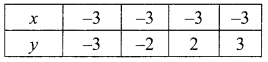Plot the points (-3, -3), (-3, -2), (-3, 2) and (-3, 3) in the graph sheet
y = 3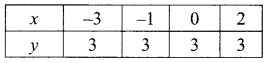Plot the points (-3, 3), (-1, 3), (0, 3) and (2, 3) in the same graph sheet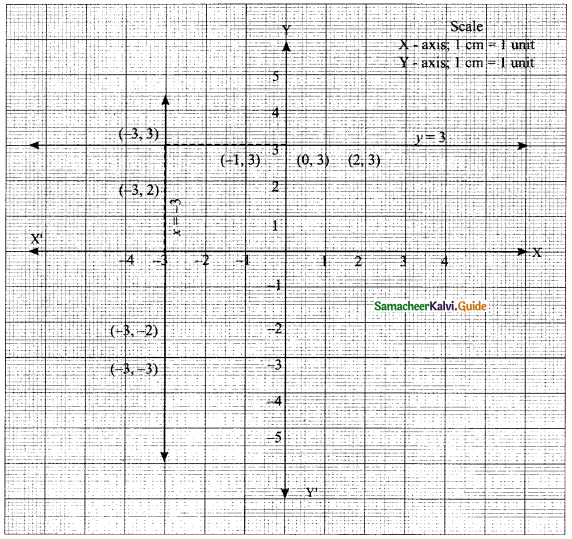The two lines l1 and l2 intersect at (-3, 3)
∴ The solution set is (-3, 3)Question 3.
Two cars are 100 miles apart. If they drive towards each other they will meet in 1 hour. If they drive in the same direction they will meet in 2 hours. Find their speed by using graphical method.
Solution:
Let the speed of the two cars be “x” and “y”.
By the given first condition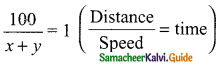x+ y = 100 → (1)
(They travel in opposite direction)
By the given second condition.
\frac{100}{x-y}= 2 [time taken in 2 hours in the same direction]
2x – 2y = 100
x – y = 50 → (2)
x + y = 100
y = 100 – x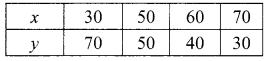Plot the points (30, 70), (50, 50), (60, 40) and (70,30) in the graph sheet
x – y = 50
-y = -x + 50
y = x – 50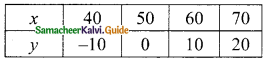Plot the points (40, -10), (50, 0), (60, 10) and (70, 20) in the same graph sheet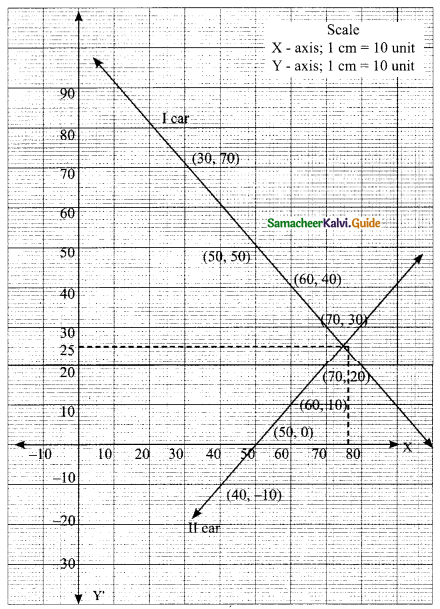The two cars intersect at (75, 25)
The speed of the first car 75 km/hr
The speed of the second car 25 km/hr